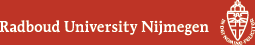## Geometry Seminar - Abstracts

### Talk

Tuesday 8 March 2022, 16:00-17:00 in HG03.082
Ben Moonen (RU)
The Coleman-Oort Conjecture

### Abstract

A long-standing conjecture of Coleman says that if we fix a genus $$g > 3$$, there are only finitely many complex curves $$C$$ of genus $$g$$, up to isomorphism, for which the Jacobian $$\mathrm{Jac}(C)$$ is an abelian variety of CM type - read this as: having a very large ring of endomorphisms. (If you prefer, replace 'curve' by 'compact Riemann surface'; it makes no difference.) I will explain why this is known to be false if $$3 < g < 8$$ and completely open otherwise, and why this is equivalent to another conjecture (Coleman-Oort) about subvarieties of moduli spaces. The mathematics that goes into this comes from many sides: from topology and differential geometry, Lie theory, number theory and transcendence theory, algebraic geometry in characteristic $$p$$, etc.; but I'll keep the talk as elementary as possible.

(Back to Geometry Seminar schedule)